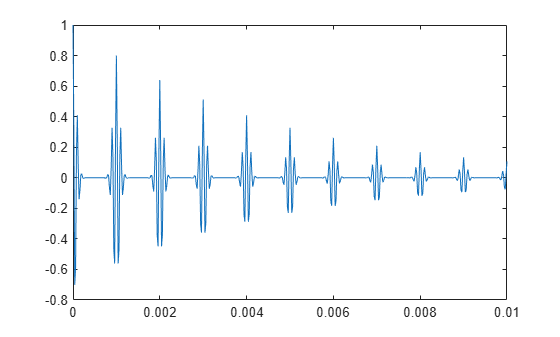The pulstran Function

The pulstran function generates pulse trains from either continuous or sampled prototype pulses. This example generates a pulse train consisting of the sum of multiple delayed interpolations of a Gaussian pulse.

The pulse train is defined to have a sample rate of 50 kHz, a pulse train length of 10 ms, and a pulse repetition rate of 1 kHz. T specifies the time instants at which the pulse train is sampled. D specifies the delay to each pulse repetition in the first column and an optional attenuation for each repetition in the second column. To construct the pulse train, pass the name of the gauspuls function to pulstran, along with additional parameters that specify a 10 kHz Gaussian pulse with 50% bandwidth.

T = 0:1/50e3:10e-3;
D = [0:1/1e3:10e-3;0.8.^(0:10)]';

Y = pulstran(T,D,'gauspuls',10e3,0.5);

plot(T,Y)See Compute Envelope Spectrum of Vibration Signal for an example that uses the pulstran function to generate vibration data for bearing analysis.